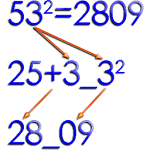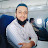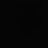# Math Tricks APK

Math Tricks

## Math Tricks description:

This program is designed for those who want to learn interesting mathematical tricks to speed up the calculating. These tricks will help solve part of the mathematical problems and tasks much faster than classical. Will also be helpful to those who want to hone basics such as the multiplication table.

When you learn these mathematical tricks, you'll be able to show off your skills to friends and prove to them that you have a talent for mathematics. New skills you can use in the store, at school, at college, at work - wherever thanks to quick calculation skills can save a lot of precious time.

Fun Math Tricks:
2. Subtraction
3. Multiplication table
4. Division
5. Multiply two digit number by 11
6. Square numbers ending in 5
7. Multiply by 5
8. Multiply by 9
9. Multiply by 4
10. Dividing by 5
11. Subtracting from 1000
12. Tough multiplication
13. Power of two
14. Adding numbers close to hundreds
15. Subtracting numbers close to hundreds
16. Multiply numbers between 11 and 19
17. Square numbers between 11 and 99
18. Multiply two digit numbers having same tens digit and ones digits add up to ten
19. Square numbers between 50 and 59
20. Square numbers between 40 and 49
21. Multiply two digit numbers editing in 1
22. Percent
23. Square numbers between 100 and 109
...
84. n^9

Training mode:
- 15 levels for every trick
- timer 15 seconds
Rating stars:
- score > 000 - ★☆☆
- score > 350 - ★★☆
- score > 650 - ★★★

The app uses the following APIs and libraries:
* MPAndroidChart - created by Philipp Jahoda
* ColorPIcker - created by Piotr Adamus
User reviewsSyed Afzal Ali

I have been using this app for last 3 years and I have also recently surpassed 20k levels too. ???? Just one thing I would like to add in this app is that it in my and many handsets like mine having virtual home keys, keys covers the down area while playing and thus not giving full view to it. I would rather rate this app 5 star if u make the full screen view available.I am using it for 2 years and this app worked so well all the time.. It improved not only my calculations but made me know many short cuts which can be used to save time. Unlike most of the apps it is not liable to the bulk of adds as well as it never stops in between as well.Simon Lee

A really great app, nearly perfect. However, accidentally pressing the Back button ends the game. It would be good if the app confirms if I want to Quit instead.Chris Anning

Good app and great for learning. It's the best Math app I've managed too find. It has all the variables I need. I particularly like the non timed math section.#Nuclear Hunters

Amazing app...the developer should given a prize for such cool options available..... we can check perday progress through graph....there are lots of modes available to go through maths practice.....I am a big fan of mathematics and I loved this game.....and once again thanks bro for this app